# Hibernate入门之主键生成策略详解

## 主键生成策略

JPA规范支持4种不同的主键生成策略（AUTO、IDENTITY、SEQUENCE、TABLE），这些策略以编程方式生成主键值或使用数据库功能（例如自动递增或序列），我们只需将@GeneratedValue注解添加到主键属性上并选择对应的生成策略。

### GenerationType.AUTO

@Entity
public class Student {

@Id
@GeneratedValue(strategy = GenerationType.AUTO)
private int id;
}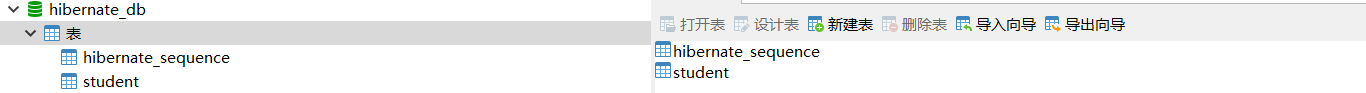insert into hibernate_sequence values ( 1 )

select next_val as id_val from hibernate_sequence for update

update hibernate_sequence set next_val= ? where next_val=?

insert into Student (email, firstName, lastName, id) values (?, ?, ?, ?)

### GenerationType.SEQUENCE

@Entity
public class Student {

@Id
@GeneratedValue(strategy = GenerationType.SEQUENCE)
private int id;
}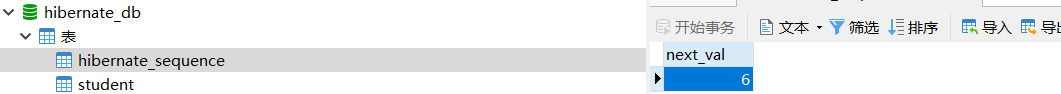@Entity
public class Student {

@Id
@GeneratedValue(strategy = GenerationType.SEQUENCE, generator = "student_generator")
@SequenceGenerator(name = "student_generator", sequenceName = "student_seq")
private int id;
}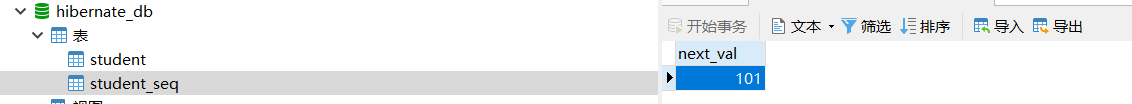@Entity
public class Student {

@Id
@GeneratedValue(strategy = GenerationType.SEQUENCE, generator = "student_seq")

private int id;
}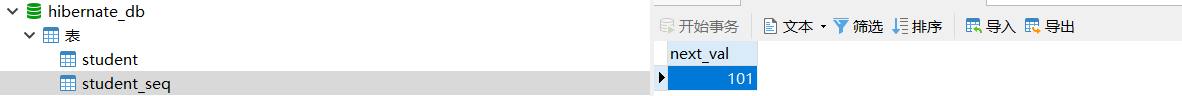@Entity
public class Student {

@Id
@GeneratedValue(strategy = GenerationType.SEQUENCE, generator = "student_generator")
@SequenceGenerator(name = "student_generator",sequenceName = "student_seq", allocationSize = 10)
private int id;
}

insert into student_seq values ( 1 )

select next_val as id_val from student_seq for update

update student_seq set next_val= ? where next_val=?

select next_val as id_val from student_seq for update

update student_seq set next_val= ? where next_val=?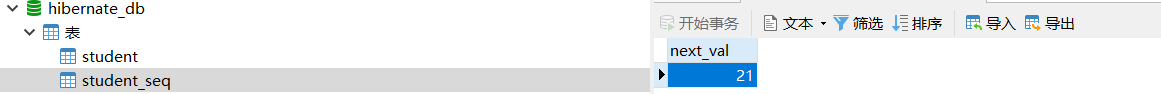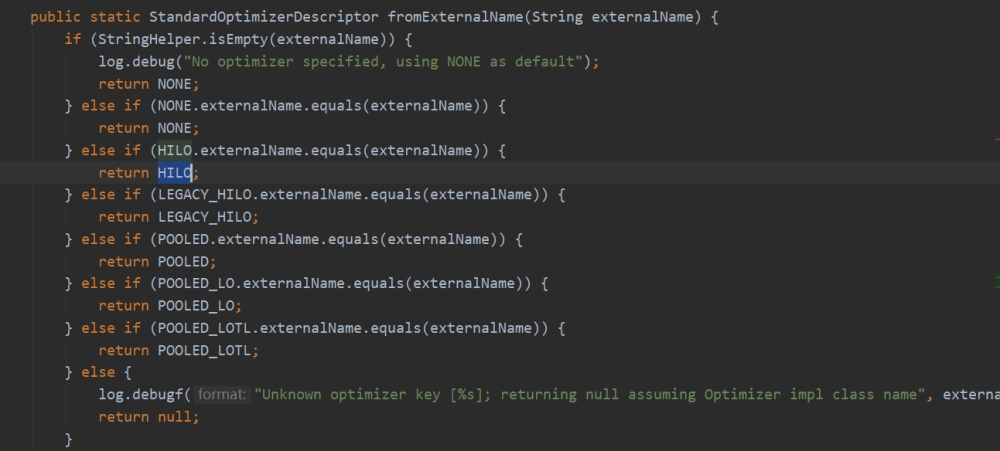@Entity
public class Student {

@Id
@GeneratedValue(strategy = GenerationType.SEQUENCE)

private int id;
}

@Entity
public class Student {

@Id
@GeneratedValue(strategy = GenerationType.SEQUENCE, generator = "student_seq")

}

@Entity
public class Student {

@Id
@GeneratedValue(strategy = GenerationType.SEQUENCE, generator = "student_generator")
@SequenceGenerator(name = "student_generator",sequenceName = "student_seq",allocationSize = 3)
}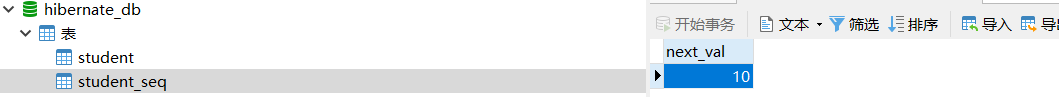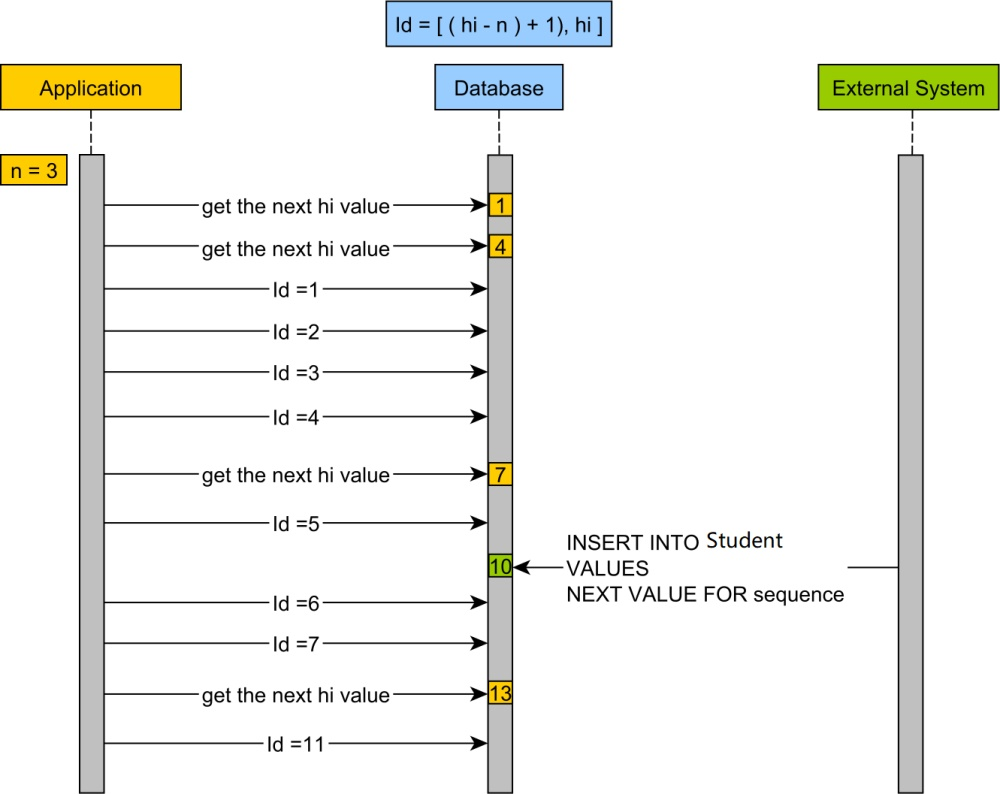@Entity
public class Student {

@Id
@GeneratedValue(strategy = GenerationType.SEQUENCE, generator = "student_seq")
@GenericGenerator(
name = "student_seq",
strategy = "sequence",
parameters = {
@Parameter(name = "sequence_name",  value = "student_seq"),
@Parameter(name = "initial_value",  value = "1"),
@Parameter(name = "increment_size",  value = "3"),
@Parameter(name = "optimizer", value = "hilo")
}
)

private int id;
}

@Entity
public class Student {

@Id
@GeneratedValue(strategy = GenerationType.SEQUENCE, generator = "student.seq")

private int id;
}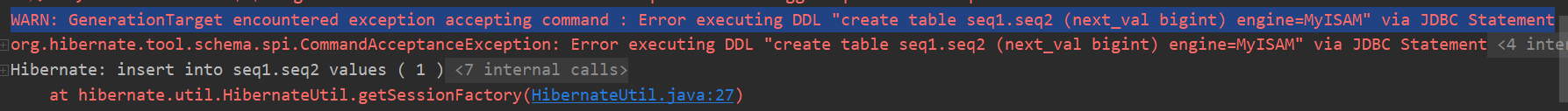### GenerationType.IDENTITY

@Entity
public class Student {

@Id
@GeneratedValue(strategy = GenerationType.IDENTITY)

private int id;
}

### GenerationType.TABLE

@Entity
public class Student {

@Id
@GeneratedValue(strategy = GenerationType.TABLE,generator = "student_generator")
@TableGenerator(name="student_generator", table="id_generator")

private int id;
}

## 总结

posted @ 2020-02-29 22:37  Jeffcky  阅读(1532)  评论(0编辑  收藏  举报